Publicité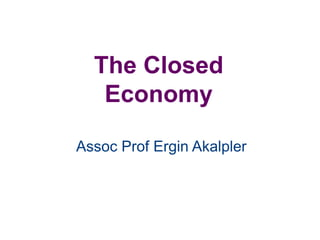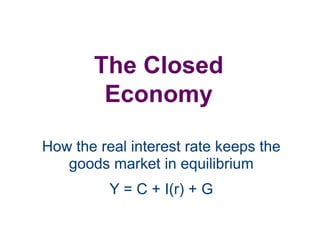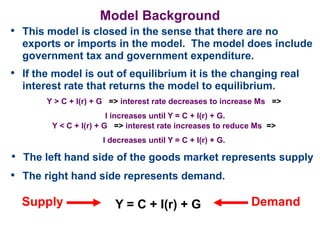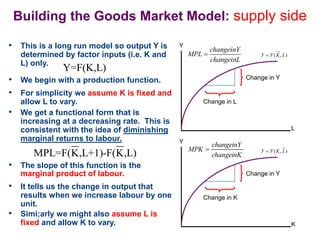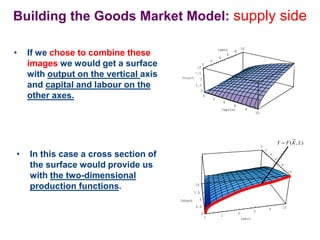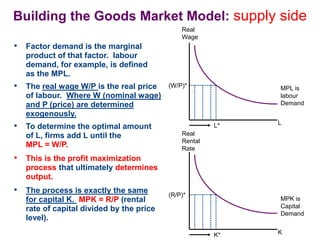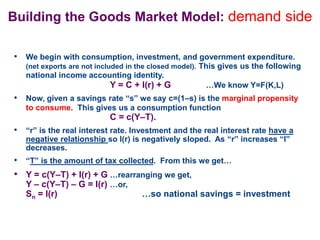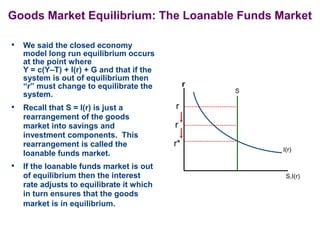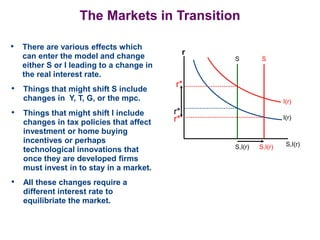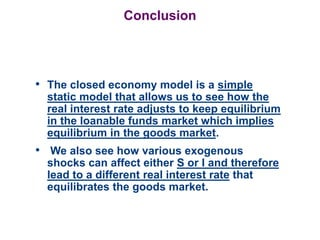1 sur 10
Publicité

### CH 1.3 macro2_Closed_Economy.ppt

1. The Closed Economy Assoc Prof Ergin Akalpler
2. The Closed Economy How the real interest rate keeps the goods market in equilibrium Y = C + I(r) + G
3. Model Background • This model is closed in the sense that there are no exports or imports in the model. The model does include government tax and government expenditure. • If the model is out of equilibrium it is the changing real interest rate that returns the model to equilibrium. Y > C + I(r) + G => interest rate decreases to increase Ms => I increases until Y = C + I(r) + G. Y = C + I(r) + G • The left hand side of the goods market represents supply • The right hand side represents demand. Y < C + I(r) + G => interest rate increases to reduce Ms => I decreases until Y = C + I(r) + G. Supply Demand
4. Building the Goods Market Model: supply side • This is a long run model so output Y is determined by factor inputs (i.e. K and L) only. MPL=F(K,L+1)-F(K,L) Y=F(K,L) L Y ( , ) Y F K L  Change in Y Change in L changeinY MPL changeinL  K Y ( , ) Y F K L  Change in Y Change in K • We begin with a production function. • For simplicity we assume K is fixed and allow L to vary. • The slope of this function is the marginal product of labour. • It tells us the change in output that results when we increase labour by one unit. • Simi;arly we might also assume L is fixed and allow K to vary. • We get a functional form that is increasing at a decreasing rate. This is consistent with the idea of diminishing marginal returns to labour. changeinK changeinY MPK 
5. 0 2 4 6 8 10 0 2 4 6 8 10 Labor 0 2.5 5 7.5 10 Output 0 2.5 5 7.5 10 Output • If we chose to combine these images we would get a surface with output on the vertical axis and capital and labour on the other axes. Building the Goods Market Model: supply side 0 2 4 6 8 10 Capital 0 2 4 6 8 10 Labor 0 2.5 5 7.5 10 Output 0 2 4 6 8 10 Capital 0 2 4 6 8 10 Labor ( , ) Y F K L  • In this case a cross section of the surface would provide us with the two-dimensional production functions.
6. Building the Goods Market Model: supply side • Factor demand is the marginal product of that factor. labour demand, for example, is defined as the MPL. MPL is labour Demand (W/P)* L* Real Wage L (R/P)* K* Real Rental Rate K MPK is Capital Demand • The real wage W/P is the real price of labour. Where W (nominal wage) and P (price) are determined exogenously. • To determine the optimal amount of L, firms add L until the MPL = W/P. • This is the profit maximization process that ultimately determines output. • The process is exactly the same for capital K. MPK = R/P (rental rate of capital divided by the price level).
7. Building the Goods Market Model: demand side • We begin with consumption, investment, and government expenditure. (net exports are not included in the closed model). This gives us the following national income accounting identity. Y = C + I(r) + G …We know Y=F(K,L) • Now, given a savings rate “s” we say c=(1–s) is the marginal propensity to consume. This gives us a consumption function C = c(Y–T). • “r” is the real interest rate. Investment and the real interest rate have a negative relationship so I(r) is negatively sloped. As “r” increases “I” decreases. • “T” is the amount of tax collected. From this we get… • Y = c(Y–T) + I(r) + G …rearranging we get, Y – c(Y–T) – G = I(r) …or, Sn = I(r) …so national savings = investment
8. Goods Market Equilibrium: The Loanable Funds Market • We said the closed economy model long run equilibrium occurs at the point where Y = c(Y–T) + I(r) + G and that if the system is out of equilibrium then “r” must change to equilibrate the system. S,I(r) r r* S I(r) r r • Recall that S = I(r) is just a rearrangement of the goods market into savings and investment components. This rearrangement is called the loanable funds market. • If the loanable funds market is out of equilibrium then the interest rate adjusts to equilibrate it which in turn ensures that the goods market is in equilibrium.
9. The Markets in Transition • There are various effects which can enter the model and change either S or I leading to a change in the real interest rate. • Things that might shift S include changes in Y, T, G, or the mpc. • Things that might shift I include changes in tax policies that affect investment or home buying incentives or perhaps technological innovations that once they are developed firms must invest in to stay in a market. S,I(r) r r* S I(r) r* r* I(r) S S,I(r) S,I(r) • All these changes require a different interest rate to equilibriate the market.
10. Conclusion • The closed economy model is a simple static model that allows us to see how the real interest rate adjusts to keep equilibrium in the loanable funds market which implies equilibrium in the goods market. • We also see how various exogenous shocks can affect either S or I and therefore lead to a different real interest rate that equilibrates the goods market.
Publicité